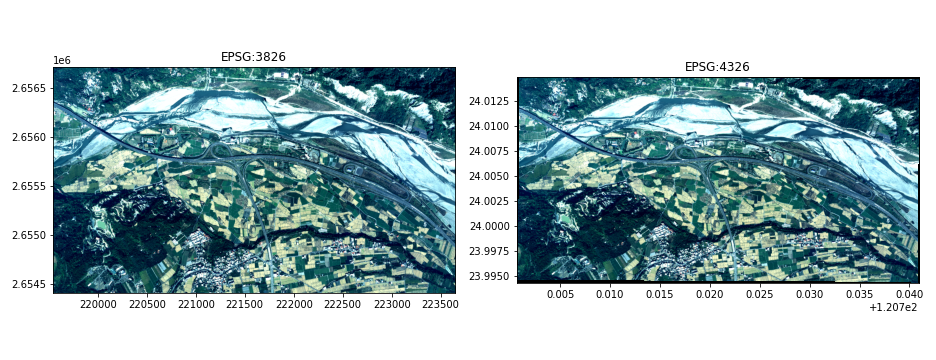# TronGisPy：GIS網格資料處理工具# 簡介TronGisPy

TronGisPy是基於Python語言，並以gdal為底層進行開發的gis影像自動化處理開源套件，同時也提供機器學習前處裡與後處理的重要功能.為了加速資料處理的開發速度與建置gis自動化流程，TronGisPy簡化了Gdal的介面，並提供各式方便的Python介面，以利工程師快速開發出有價值且可自動化的應用.由於TronGIS Py的所有函式幾乎是基於解決實際問題而進行開發的，且已經至少十個大小不同的專案，因此應該已經可以滿足大部分GIS專案的需求.本篇文章主要在介紹TronGIS Py起源、模組、安裝方法以及基礎的GIS影像觀念，應用方式及程式碼會在後面文章陸續介紹.

# 模組簡介

• 光栅：儲存tif裡所有資訊的物件模組，其中資訊包含影像本身(光栅数据)、投影座標(光栅投影)、影像位置解析度與旋轉角(光栅.geo_Transform)、同時亦有繪圖(光栅.Plot())、轉投影(光栅.reproject())與從新定位網格位置(光栅.remap())或解析度(光栅.Refine_Resolsion())等功能。
• ShapeGrid：網格資料與向量資料的轉換功能模組。本模組可以方便地將網格資料向量化(矢量化_图层)、將向量資料網格化(栅格化_图层)、或透過已知的光栅網格將向量資料網格化(栅格化_图层_by_REF_RASTER)、剪裁多边形光栅(或使用_對網格進行切割_WITH_POLYGON)。
• 插值：影像補遺的功能模組。此模組可以透過反距離加權法(Idw)或像素鄰近的數值將遺漏值填上.可以選擇使用像鄰近網格的平均值(Mean_Interpolation)或是眾數(多数数内插)進行內差，亦可使用Gdal實作的反距離加權法(GDAL_FILNODATA)進行內差。其中為了加速像素鄰近網格过滤(Sequoia的卷機運算，相關函式皆有使用Numba進行平行化運算.)
• crs：協助座標系統轉換的功能模組。經緯度座標與Numpy.array的網格索引的轉換(COORDS_TO_NPIDXS，NPIDXS_TO_COORDS)、投影座標格式wkt與epsg的轉換(epsg_to_wkt，wkt_to_epsg)。
• 规格化器：像素數值規一化的物件模組。由於gis影像感測器繁多像素數值型態有Float、8位或16位，此模組可以協助將不同的像素數值型態進行規一化(Normalizer.fit_Transform())。另外，由於許地理信息系统影像感光元件較敏感或無法確保足夠的光源，在程現時常會有亮度太暗的狀況，此時可以將像素數值中的離群值切掉(Normalizer.Clip_by_Percentage())，以利影像呈現與觀察。
• SplittedImage：協助裁切與組合地理信息系统影像的物件模組。由於地理信息系统影像常常非常大張(例如10000×10000像素)，如果直接放入機器學習模型，一方面資料量可能不足，另一方面也會造成記憶體與運算量的龐大負擔.因此透過拆分图像物件可以把影像按照移動窗格的模式進行切割，待機器學習模型判釋完成，再將判釋結果組合(GET_COMPLIED_IMAGE)為與原影像具有相同空間資訊的影像。
• 类型预测：協助Numpy與gdal資料格式轉換的功能模組。主要提供gdal資料格式轉換為ndpy資料格式的函式(tgp.gdaldtype_to_npdtype)、與numpy資料格式的函式轉換為gdal資料格式的函式(tgp.npdtype_to_gdaldtype)。

# 安裝

• 透過Docker直接安裝：Docker Pull Jeremy4555/trongispy：Latest，並透過Docker Run-It–RM Jeremy4555/trongispy：最新/bin/bash啟動Docker，或請見TronGisPy的Docker文件。
• Windows建議直接安裝別人Build好的套件。
• LINUX的部分請注意gdal必須先安裝到可以透過gdal_info–Version取得gdal 3.0.4，Release 2020/01/28後，再透過pip安裝pip install gdal=3.0.4(安裝gdal的dockerfile)，務必確認gdal_info的版本與python中的gdal版本必須一致，其餘套件則透過pip安裝即可。

GDAL==3.0.4Fiona==1.8.13Shapely==1.6.4.post2geopandas==0.7.0Rtree==0.9.4opencv_python==4.1.2

pip install TronGisPy

# GIS影像的屬性與基本操作

import TronGisPy as tgpimg_fp = tgp.get_testing_fp('aereo_tif') # 取得測試資料的路徑ras = tgp.read_raster(img_fp) # 讀取GIS影像ras.plot(clip_percentage=(0.1, 0.9)) # 把影像數值前後10%切掉增顯並畫出影像## 数据(影像數值)

import TronGisPy as tgpfp = tgp.get_testing_fp('aereo_tif') # 取得測試資料的路徑ras = tgp.read_raster(fp) # 讀取GIS影像RED, NIR = ras.data[:, :, 0], ras.data[:, :, 3] # 取得紅光跟近紅外光NDVI = (NIR - RED) / (NIR + RED + 10**-6) # 計算NDVIras_NDVI = ras.copy() # 複製原本GIS影像的地理資訊ras_NDVI.data = NDVI # 複製將數值資料改為NDVIras_NDVI.plot(clip_percentage=(0.1, 0.9), cmap='gray') # 把影像數值前後10%切掉增顯並畫出影像>>> import gdal>>> print([func for func in dir(gdal) if "GDT" in func])['GDT_Byte', 'GDT_CFloat32', 'GDT_CFloat64', 'GDT_CInt16', 'GDT_CInt32', 'GDT_Float32', 'GDT_Float64', 'GDT_Int16', 'GDT_Int32', 'GDT_TypeCount', 'GDT_UInt16', 'GDT_UInt32', 'GDT_Unknown']

## 投影(投影座標)

>>> import TronGisPy as tgp>>> fp = tgp.get_testing_fp('aereo_tif')  # 取得測試資料的路徑>>> ras = tgp.read_raster(fp) # 讀取GIS影像>>> print("Projection(WKT):", ras.projection)  # 取得投影座標WKTProjection(WKT): PROJCS["TWD97 / TM2 zone 121",GEOGCS["TWD97",DATUM["Taiwan_Datum_1997",SPHEROID["GRS 1980",6378137,298.257222101,AUTHORITY["EPSG","7019"]],AUTHORITY["EPSG","1026"]],PRIMEM["Greenwich",0,AUTHORITY["EPSG","8901"]],UNIT["degree",0.0174532925199433,AUTHORITY["EPSG","9122"]],AUTHORITY["EPSG","3824"]],PROJECTION["Transverse_Mercator"],PARAMETER["latitude_of_origin",0],PARAMETER["central_meridian",121],PARAMETER["scale_factor",0.9999],PARAMETER["false_easting",250000],PARAMETER["false_northing",0],UNIT["metre",1,AUTHORITY["EPSG","9001"]],AXIS["Easting",EAST],AXIS["Northing",NORTH],AUTHORITY["EPSG","3826"]]>>> print("Projection(EPSG):", tgp.wkt_to_epsg(ras.projection))  # 取得投影座標WKTProjection(EPSG): 3826>>> print("EPSG(4326) to WKT:", tgp.epsg_to_wkt(4326))  # 取得投影座標WKTEPSG(4326) to WKT: GEOGCRS["WGS 84",DATUM["World Geodetic System 1984",ELLIPSOID["WGS 84",6378137,298.257223563,LENGTHUNIT["metre",1]]],PRIMEM["Greenwich",0,ANGLEUNIT["degree",0.0174532925199433]],CS[ellipsoidal,2],AXIS["geodetic latitude (Lat)",north,ORDER,ANGLEUNIT["degree",0.0174532925199433]],AXIS["geodetic longitude (Lon)",east,ORDER,ANGLEUNIT["degree",0.0174532925199433]],USAGE[SCOPE["unknown"],AREA["World"],BBOX[-90,-180,90,180]],ID["EPSG",4326]]

import TronGisPy as tgpfrom matplotlib import pyplot as pltras = tgp.read_raster(tgp.get_testing_fp('aereo_tif')) # 讀取GIS影像ras_reproj = ras.reproject(dst_crs='EPSG:4326') # 轉投影到EPSG:4326fig, (ax1, ax2) = plt.subplots(1, 2, figsize=(13, 5)) # 開啟一張1x2網格的畫布ras.plot(ax=ax1, clip_percentage=(0.1, 0.9)) # 畫上EPSG:3826的圖像ax1.set_title('EPSG:3826')ras_reproj.plot(ax=ax2, clip_percentage=(0.1, 0.9)) # 畫上EPSG:4326的圖像ax2.set_title('EPSG:4326')plt.show()## 地理运输(起始位置、網格解析度以及旋轉角)

GeoTransform是由六個數字所構成，如下面程式碼示範如何透過TronGisPy取得GeoTransform。

>>> import TronGisPy as tgp>>>ras = tgp.read_raster(tgp.get_testing_fp('aereo_tif')) # 讀取GIS影像>>> ras.geo_transform(219541.78148359052, 0.0201129761884431, 2.3639991549296866, 2654444.8101615803, 2.3639991549296155, -0.020112976188556786)

# 將直從geo_transform取出c, a, b, f, d, e = geo_transform

import TronGisPy as tgpras = tgp.read_raster(tgp.get_testing_fp('aereo_tif')) # 讀取GIS影像npidxs_col, npidxs_row = np.meshgrid(range(ras.cols), range(ras.rows)) # 給每個像素都取得row_idx跟col_idxnpidxs = np.stack([npidxs_row, npidxs_col], axis=-1).reshape(-1, 2) # 將每個像素的row_idx跟col_idx整理在同一筆資料coords = tgp.npidxs_to_coords(npidxs, ras.geo_transform) # 將影像像素索引值轉換為座標位置for npidx, coord in zip(npidxs[:5], coords[:5]): # 印出頭五個結果    print(npidx, "=>", coord)

import TronGisPy as tgpimport geopandas as gpdfrom matplotlib import pyplot as pltras = tgp.read_raster(tgp.get_testing_fp('aereo_tif')) # 讀取GIS影像df = gpd.read_file(tgp.get_testing_fp('aereo_tif_clipper')) # 取得疊圖向量fig, ax = plt.subplots(1, 1)# 創建一個畫布，並把圖片跟像量化在同一畫布上ras.plot(ax=ax, clip_percentage=(0.1, 0.9)) # TronGisPy的 plot() ，已經將座標資訊考量進去，僅需與向量畫在同一畫布即可。df.loc[df['value'] == 3].boundary.plot(ax=ax, color='red')plt.show()